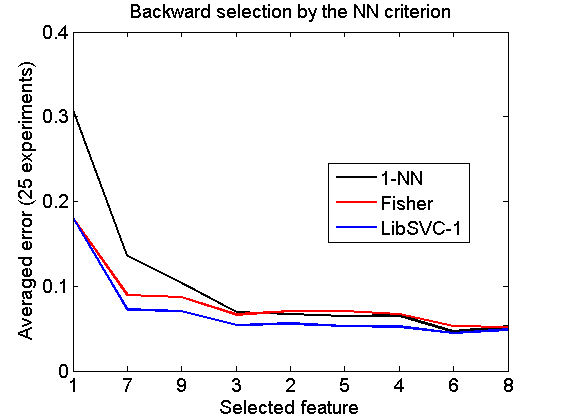# featsel_ex1

Examples of various feature selection procedures, organised per procedure

PRTools and PRDataSets should be in the path

Download the m-file from here. See http://37steps.com/prtools for more.

Feature curves are shown for 6 feature rankings computed by 3 procedures:

• Individual selection
• Forward selection
• Backward selecton

and 2 criteria:

• The Mahalanobis distance
• The leave-one-out nearest neighbor performance

These criteria are computed for the entire training set. Each of the 6 plots shows the performance of 3 classifiers based on a 50-50 random split of the dataset for training and testing.

• 1-NN rule
• The Fisher classifier
• The linear support vector machine.

In another, very similar example, featsel_ex2 the same curves are organised per classifier instead of per ranking procedure.

## Show dataset

The Breast Wisconsin dataset is based on 9 features and 683 objects in two classes of 444 and 239 objects.

```delfigs
a = breast;
a = setprior(a,0);
scattern(a*pcam(a,2));
title(['PCA projection of the ' getname(a) ' dataset'])
```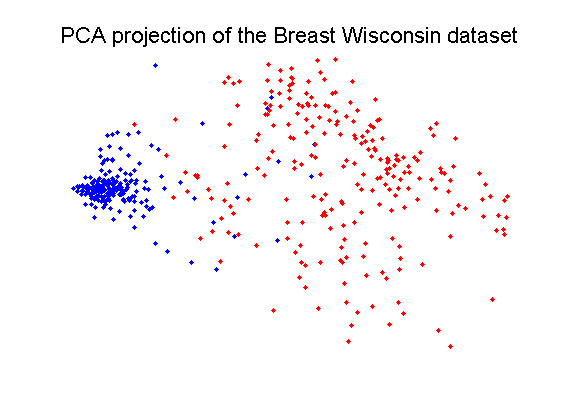## Define classifiers

```w1 = setname(knnc([],1),'1-NN');
w2 = setname(fisherc,'Fisher');
w3 = setname(libsvc,'LibSVC-1');
% number of random train-test splits used in the feature curve routine.
nreps = 25;
```

## Original feature curves

These curves are based on the feature ranking of the original dataset

```randreset; e = clevalf(a,{w1,w2,w3},[],0.5,nreps);
figure; plote(e);
title('Feature curves for original feature ranking')
xlabel('Feature number'); set(gca,'xscale','linear');
set(gca,'xticklabel',1:size(a,2)); set(gca,'xtick',1:size(a,2));
fontsize(14);
```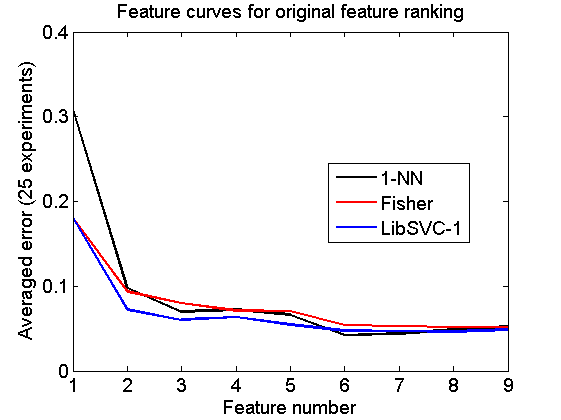## Individual selection by the Mahalanobis criterion

```v = featseli(a,'maha-s',size(a,2));
randreset; e = clevalf(a*v,{w1,w2,w3},[],0.5,nreps);
figure;  plote(e);
title('Individual selection by the Mahalanobis criterion')
xlabel('Selected feature'); set(gca,'xscale','linear');
set(gca,'xticklabel',+v); set(gca,'xtick',1:size(a,2));
fontsize(14);
```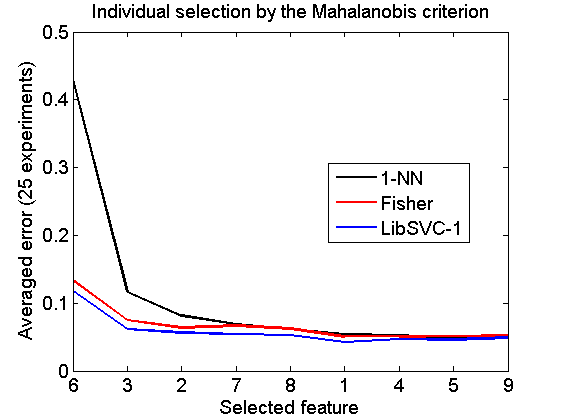## Forward selection by the Mahalanobis criterion

```v = featself(a,'maha-s',size(a,2));
randreset; e = clevalf(a*v,{w1,w2,w3},[],0.5,nreps);
figure;  plote(e);
title('Forward selection by the Mahalanobis criterion')
xlabel('Selected feature'); set(gca,'xscale','linear');
set(gca,'xticklabel',+v); set(gca,'xtick',1:size(a,2));
fontsize(14);
```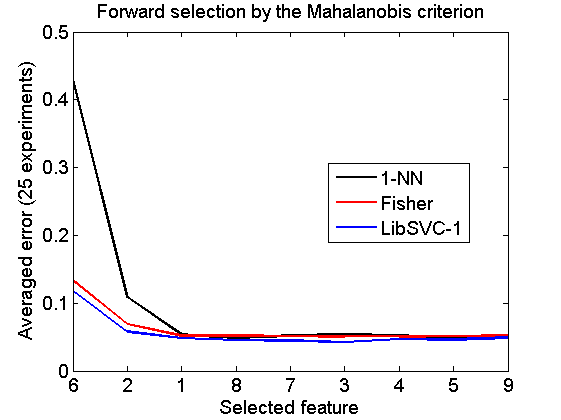## Backward selection by the Mahalanobis criterion

```[v,r] = featselb(a,'maha-s',1);
% the next statment is needed to retrieve the feature ranking
v = featsel(size(a,2),[+v abs(r(2:end,3))']);
randreset; e = clevalf(a*v,{w1,w2,w3},[],0.5,nreps);
figure;  plote(e);
title('Backward selection by the Mahalanobis criterion')
xlabel('Selected feature'); set(gca,'xscale','linear');
set(gca,'xticklabel',+v); set(gca,'xtick',1:size(a,2));
fontsize(14);
```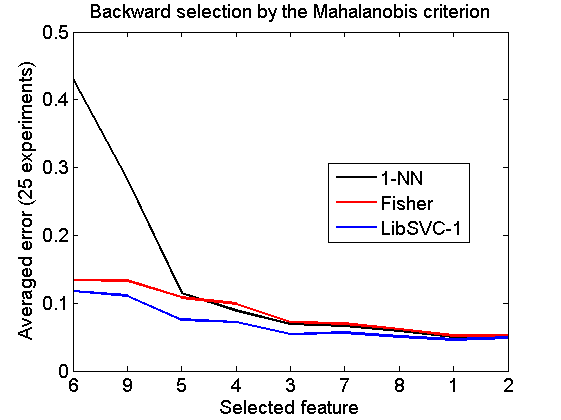## Individual selection by the NN criterion

```v = featseli(a,'NN',size(a,2));
randreset; e = clevalf(a*v,{w1,w2,w3},[],0.5,nreps);
figure;  plote(e);
title('Individual selection by the NN criterion')
xlabel('Selected feature'); set(gca,'xscale','linear');
set(gca,'xticklabel',+v); set(gca,'xtick',1:size(a,2));
fontsize(14);
```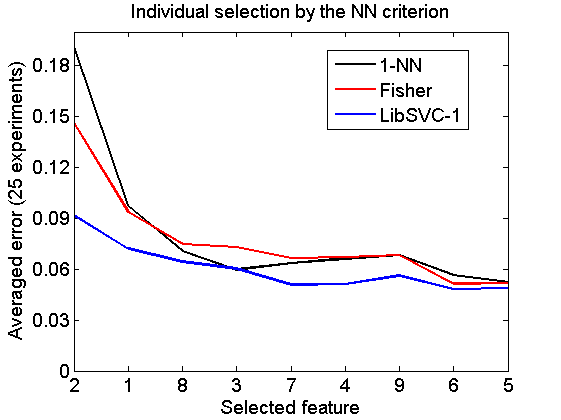## Forward selection by the NN criterion

```v = featself(a,'NN',size(a,2));
randreset; e = clevalf(a*v,{w1,w2,w3},[],0.5,nreps);
figure;  plote(e);
title('Forward selection by the NN criterion')
xlabel('Selected feature'); set(gca,'xscale','linear');
set(gca,'xticklabel',+v); set(gca,'xtick',1:size(a,2));
fontsize(14);
```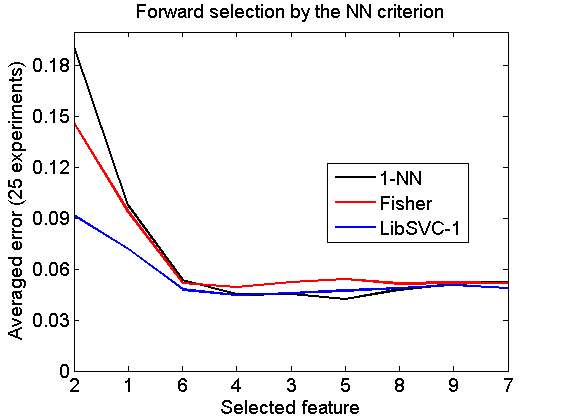## Backward selection by the NN criterion

```[v,r] = featselb(a,'NN',1);
% the next statment is needed to retrieve the feature ranking
v = featsel(size(a,2),[+v abs(r(2:end,3))']);
randreset; e = clevalf(a*v,{w1,w2,w3},[],0.5,nreps);
figure;  plote(e);
title('Backward selection by the NN criterion')
xlabel('Selected feature'); set(gca,'xscale','linear');
set(gca,'xticklabel',+v); set(gca,'xtick',1:size(a,2));
fontsize(14);
```